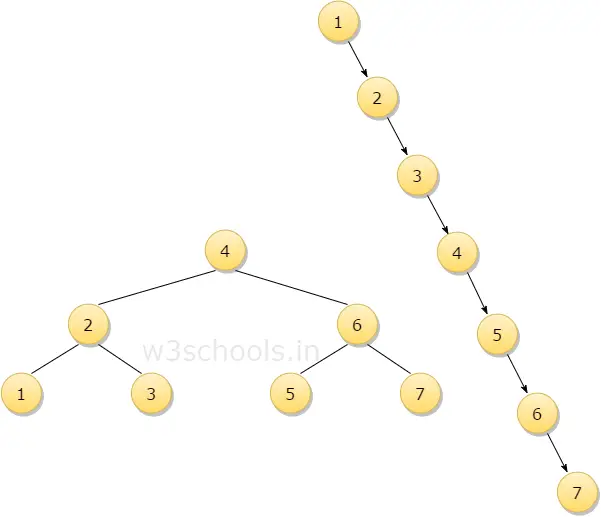## Data Structure Tutorial Index

The tree is one of the most essential data structures used to efficiently perform operations like insertion, deletion, and search of values. However, while working with a large volume of data, constructing a well-balanced tree for sorting all data is not feasible. Thus only valuable data is stored as a tree, and the actual volume of data being used continually changes through the insertion of new data and deletion of existing data. In some cases, the NULL link to a binary tree to special links is called a thread; hence, it is possible to perform traversals, insertions, and deletions without using either stack or recursion. In this tutorial, you will learn about the Height balanced tree, also known as the AVL tree.

## What is The AVL Tree?

AVL tree is a binary search tree in which the difference of heights of left and right subtrees of any node is less than or equal to one. The technique of balancing the height of binary trees was developed by Adelson, Velskii, and Landi and hence given the short form as AVL tree or Balanced Binary Tree.

An AVL tree can be defined as follows:

Let T be a non-empty binary tree with TL and TR as its left and right subtrees. The tree is height balanced if:

• TL and TR are height balanced
• hL - hR <= 1, where hL - hR are the heights of TL and TR

The Balance factor of a node in a binary tree can have values 1, -1, or 0, depending on whether the height of its left subtree is greater, less than, or equal to the height of the right subtree.

Since AVL trees are height balanced, operations like insertion and deletion have low time complexity. Let us consider an example:

If you have the following tree having keys 1, 2, 3, 4, 5, 6, and 7 then the binary tree will be like the second figure:The algorithm requires seven comparisons to insert a node with a key Q in the binary tree, but if you insert the same key in the AVL tree, from the above 1st figure, you can see that the algorithm will require three comparisons.

## Representation of AVL Trees

``````Struct AVLNode
{
int data;
struct AVLNode *left, *right;
int balfactor;
};
``````

## Algorithm for different Operations on AVL

### For Insertion:

Step 1: Insert a new element into the tree using BST's (Binary Search Tree) insertion logic.

Step 2: After inserting the elements, you have to check the Balance Factor of each node.

Step 3: When every node's balance factor is 0 or 1, or -1, the algorithm will proceed to the next operation.

Step 4: When the balance factor of any node comes other than the above three values, the tree is said to be imbalanced. Then perform the suitable Rotation to make it balanced, and the algorithm will proceed to the next operation.

### For Deletion:

Step 1: Firstly, find that node where k is stored

Step 2: Secondly, delete those contents of the node (Suppose the node is x)

Step 3: Claim: Deleting a node in an AVL tree can be reduced by deleting a leaf. There are three possible cases:

• When x has no children, then delete x
• When x has one child, let x' becomes the child of x.
• Notice: x' cannot have a child since subtrees of T can differ in height by at most one :
• then replace the contents of x with the contents of x'
• then delete x' (a leaf)
• Step 4:  When x has two children,
• then find x's successor z (which has no left child)
• then replace x's contents with z's contents, and
• delete z

In all three cases, you will end up removing a leaf.